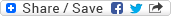## Wednesday, June 11, 2008

### Puzzle: What's the no. of trailing zeroes in 100!

Puzzle: What's the number of trailing zeroes in 100!

Solution: FYI - 100! = 1 * 2 * 3 * .... * 99 * 100

Approach: 1 trailing zero will be produced by every different 10 as a factor in the product. One 10 will be produced by one 5 and one 2. Since, number of 2s will always be more than number of 5s in the overall product, so we'll go by counting the occurrences of 5s to compute the number of occurrences of 10s as factors. Similarly, counting the occurrences of 5*5 = 25 will give us the total number of occurrences of 100s in the product... we need to calculate this as one 100 will contribute 2 zeroes at the end of the product. 1 out of these 2 zeroes would have already been counted by calculating the number of occurrences of 10s and the second zero will be counted by calculating the number of occurrences of 100s. Right? We don't need to continue the same way for finding the occurrences of 1000s as all such cases would have already been counted either by counting the occurrences of 10s or by counting the occurrences of 100s. Okay... let's try to understand this. One possible combination of factors which can produce 1000 is (25, 40). But, we have already counted the occurrences of 25 and that of 5. So, this pair is already covered. We can easily see the same happening for any other possible combination of factors which can produce 1000. So, the rule of thumb is that we continue counting the occurrences of 5, 5*5, ... till it exceeds the number whose factorial is being counted for trailing zeroes.

Answer: Hence, the number of trailing 0s in 100! = ceil(100, 5) + ceil(100, 25) = 20 + 4 = 24. Here, the ceil function gives the total number of occurrences of the second operand in the factorial of the first operand.